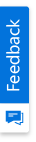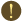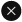We use cookies to give you the best experience on our website. If you continue to browse, then you agree to our privacy policy and cookie policy.Unfortunately, activation email could not send to your email. Please try again.# Paste only the formula value of Excel cell in C#, VB.NET

Platform: WinForms |
Control: XlsIO
Tags:

Syncfusion Excel (XlsIO) library is a .NET Excel library used to create, read, and edit Excel documents. Also, converts Excel documents to PDF files. Using this library, you can copy and paste only the formula values of cell ignoring the formula.

## Steps to paste only the formula value of cell, programmatically:

1. Create a new C# console application project.Create a new C# console application project

1. Install the Syncfusion.XlsIO.WinForms NuGet package as reference to your .NET Framework application from NuGet.org.Install NuGet package to the project

1. You can copy a range of cells to another location using the CopyTo method of IRange interface with different copy range options available under the enum ExcelCopyRangeOptions.

C#

```//Copy source range to destination range
worksheet.Range["C1:C5"].CopyTo(worksheet.Range["E1"], ExcelCopyRangeOptions.None);
```

VB.NET

```'Copy source range to destination range
worksheet.Range("C1:C5").CopyTo(worksheet.Range("E1"), ExcelCopyRangeOptions.None)
```

1. Include the following namespaces in Program.cs file.

C#

```using Syncfusion.XlsIO;
```

VB.NET

```Imports Syncfusion.XlsIO
```

1. Include the following code snippet in main method of Program.cs file to copy and paste only the formula value of cell.

C#

```using (ExcelEngine excelEngine = new ExcelEngine())
{
//Instantiate the Excel application object
IApplication application = excelEngine.Excel;

//Create a new Excel workbook
IWorkbook workbook = application.Workbooks.Create(1);

//Get the first worksheet in workbook into IWorksheet
IWorksheet worksheet = workbook.Worksheets;

//Assign data in the worksheet
worksheet.Range["A1"].Number = 1;
worksheet.Range["A2"].Number = 2;
worksheet.Range["A3"].Number = 3;
worksheet.Range["A4"].Number = 4;
worksheet.Range["A5"].Number = 5;

//Assign formulas in the worksheet
worksheet.Range["C1"].Formula = "=A1+A1";
worksheet.Range["C2"].Formula = "=A2+A2";
worksheet.Range["C3"].Formula = "=A3+A3";
worksheet.Range["C4"].Formula = "=A4+A4";
worksheet.Range["C5"].Formula = "=A5+A5";

//Copy only the formula values from source range to destination range
worksheet.Range["C1:C5"].CopyTo(worksheet.Range["E1"], ExcelCopyRangeOptions.None);

//Save the Excel document
workbook.SaveAs("Output.xlsx");
}
```

VB.NET

```Using excelEngine As ExcelEngine = New ExcelEngine()
'Instantiate the Excel application object
Dim application As IApplication = excelEngine.Excel

'Create a new Excel workbook
Dim workbook As IWorkbook = application.Workbooks.Create(1)

'Get the first worksheet in workbook into IWorksheet
Dim worksheet As IWorksheet = workbook.Worksheets(0)

'Assign data in the worksheet
worksheet.Range("A1").Number = 1
worksheet.Range("A2").Number = 2
worksheet.Range("A3").Number = 3
worksheet.Range("A4").Number = 4
worksheet.Range("A5").Number = 5

'Assign formulas in the worksheet
worksheet.Range("C1").Formula = "=A1+A1"
worksheet.Range("C2").Formula = "=A2+A2"
worksheet.Range("C3").Formula = "=A3+A3"
worksheet.Range("C4").Formula = "=A4+A4"
worksheet.Range("C5").Formula = "=A5+A5"

'Copy only the formula values from source range to destination range
worksheet.Range("C1:C5").CopyTo(worksheet.Range("E1"), ExcelCopyRangeOptions.None)

'Save the Excel document
workbook.SaveAs("Output.xlsx")
End Using
```

A complete working sample of how to copy and paste only the formula values of cells ignoring the formulas can be downloaded from Copy-Values.zip.

By executing the program, you will get the output Excel document as follows.Output Excel document

Take a moment to peruse the documentation where you will find other options like enable and disable calculation, reading and writing formula, calculated value, array of formula, incremental and external formula, named range and more.

Click here to explore the rich set of Syncfusion Excel (XlsIO) library features.

Note:

Starting with v16.2.0.x, if you reference Syncfusion assemblies from trial setup or from the NuGet feed, include a license key in your projects. Refer the link to learn about generating and registering Syncfusion license key in your application to use the components without trial message.

2X faster development

The ultimate WinForms UI toolkit to boost your development speed.You are using an outdated version of Internet Explorer that may not display all features of this and other websites. Upgrade to Internet Explorer 8 or newer for a better experience.